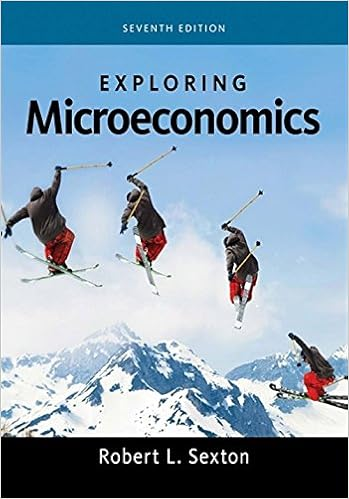# The eoq model is solved using calculus but the key

• Test Prep
• 60
• 98% (190) 187 out of 190 people found this document helpful

This preview shows page 25 - 28 out of 60 pages.

##### We have textbook solutions for you!
The document you are viewing contains questions related to this textbook.The document you are viewing contains questions related to this textbook.
Chapter 2 / Exercise 3
Exploring Economics
SextonExpert Verified
40) The EOQ model is solved using calculus but the key intuition is that relevant total costs are minimized when relevant ordering costs equal relevant carrying costs.
Diff: 2Objective: 2AACSB: Analytical thinking41) The reorder point is the quantity level of inventory at which a new purchase order is made.
Diff: 1Objective: 2AACSB: Analytical thinking
##### We have textbook solutions for you!
The document you are viewing contains questions related to this textbook.The document you are viewing contains questions related to this textbook.
Chapter 2 / Exercise 3
Exploring Economics
SextonExpert Verified
42) Due to unprecedented growth during the year, Flowers by Kelly decided to use some of its surplus cash to increase the size of several inventory order quantities that had been previously determined using an EOQ model. Required:Identify whether increasing the size of inventory orders will increase, decrease, or have no effect on each of the following items. ________a. Average inventory ________b.Cost of goods sold ________c. Number of orders per year ________d.Total annual carrying costs ________e. Total annual carrying and ordering costs ________f.Total annual ordering costs Answer: a.Increaseb.No effectc.Decreased.Increasee.Depends which costs increase/decrease moref.Decrease Diff: 2Objective: 2AACSB: Application of knowledge43) The only product of a company has an annual demand of 14,000 units. The cost of placing an order is \$70 and the cost of carrying one unit in inventory for one year is \$20. Required: Determine the economic order quantity.
Diff: 2Objective: 2AACSB: Application of knowledge
44) Picture Company has one particular product that has an annual demand of 5,000 units. Total manufacturing costs per unit total \$50. Ordering costs for the product total \$60 per purchase order. Currently, the carrying costs per unit are 25% of manufacturing costs. Required: Determine the economic manufacturing order quantity.
Diff: 2Objective: 2AACSB: Application of knowledge45) Ralph was in the process of completing the quarterly planning for the purchasing department when a major computer malfunction lost most of his data. For direct material XXX he was able to recover the following: Average inventory level of XXX200Orders per year40Average daily demand48Working days per year250Annual ordering costs\$4,000Annual carrying costs\$6,000Ralph purchases at the EOQ quantity level. Required: Determine the annual demand, the cost of placing an order, the annual carrying cost of oneunit, and the economic order quantity.
Diff: 3Objective: 2AACSB: Application of knowledge
•••